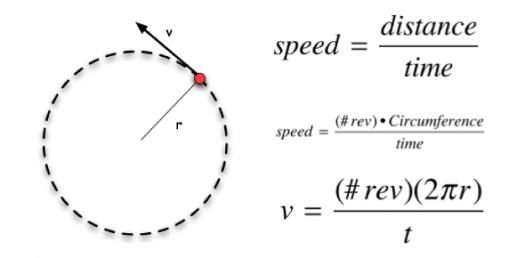# AP Physics Test: Circular Motion And Gravitation! Trivia Quiz

Approved & Edited by ProProfs Editorial Team
At ProProfs Quizzes, our dedicated in-house team of experts takes pride in their work. With a sharp eye for detail, they meticulously review each quiz. This ensures that every quiz, taken by over 100 million users, meets our standards of accuracy, clarity, and engagement.
| Written by Sfduke
S
Sfduke
Community Contributor
Quizzes Created: 57 | Total Attempts: 8,166
Questions: 5 | Attempts: 424Settings.

• 1.

### In the figure above, according to Kepler’s laws of planetary motion,

• A.

A1 = A2.

• B.

Delta t1 > delta t2.

• C.

If delta t1 = delta t2, then the orbit is circular

• D.

If delta t1 = delta t2, then A1 = A2.

D. If delta t1 = delta t2, then A1 = A2.
Explanation
According to Kepler's laws of planetary motion, the area swept out by a planet in a given time is equal for equal time intervals. This means that if delta t1 (the time taken for planet 1 to sweep out an area) is equal to delta t2 (the time taken for planet 2 to sweep out an area), then the areas A1 and A2 swept out by the two planets will also be equal. Therefore, the correct answer is "if delta t1 = delta t2, then A1 = A2."

Rate this question:

• 2.

### The equation for the speed of an object in circular orbit is to the left.  What does m represent in this equation?

• A.

The mass of the sun

• B.

The mass of Earth

• C.

The mass of the central object

• D.

The mass of the orbiting object

C. The mass of the central object
Explanation
The variable "m" in the equation represents the mass of the central object. This means that the speed of an object in a circular orbit is dependent on the mass of the central object.

Rate this question:

• 3.

### How would the speed of Earth’s orbit around the sun change if Earth’s mass increased by 4 times?

• A.

It would increase by a factor of 2.

• B.

It would increase by a factor of 4.

• C.

It would decrease by a factor of 2.

• D.

The speed would not change.

A. It would increase by a factor of 2.
Explanation
If Earth's mass increased by 4 times, according to the law of conservation of angular momentum, the product of the mass and velocity must remain constant. Since the mass has increased, the velocity must decrease in order to maintain the same angular momentum. Therefore, the speed of Earth's orbit around the sun would decrease by a factor of 2.

Rate this question:

• 4.

### How would the speed of Earth’s orbit around the sun change if Earth’s distance from the sun increased by 4 times?

• A.

It would increase by a factor of 2.

• B.

It would increase by a factor of 4.

• C.

It would decrease by a factor of 2.

• D.

The speed would not change.

C. It would decrease by a factor of 2.
Explanation
If Earth's distance from the sun increased by 4 times, the speed of Earth's orbit around the sun would decrease. This is because according to Kepler's laws of planetary motion, the speed of a planet in its orbit is inversely proportional to its distance from the sun. Therefore, if the distance increases by a factor of 4, the speed would decrease by a factor of 2.

Rate this question:

• 5.

### The law of planetary motion that relates the speed of a planet to its position in its orbit about the Sun is:

• A.

Kepler's first law

• B.

Kepler's third law

• C.

The Law of Equal Areas

• D.

The Law of InertiaBack to top